[Introduction]  [Signal arithmetic]  [Signals and noise]   [Smoothing]   [Differentiation]  [Peak Sharpening]  [Harmonic analysis]   [Fourier convolution]  [Fourier deconvolution]  [Fourier filter]  [Wavelets]   [Peak area measurement]  [Linear Least Squares]  [Multicomponent Spectroscopy]  [Iterative Curve Fitting]  [Hyperlinear quantitative absorption spectrophotometry] [Appendix and Case Studies]  [Peak Finding and Measurement]  [iPeak]   [iSignal]  [Peak Fitters]   [iFilter]  [iPower]  [List of downloadable software]  [Interactive tools]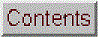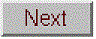## Signals and noise

[Sources]   [Measuring noise]   [Signal-to-noise ratio]  [Detection limit] [Ensemble averaging]   [Frequency spectrum]  [Dependence on signal amplitude]   [Probability distribution[Spreadsheets]   [Matlab/Octave]  [Difference between scripts and functions]  [Interactive tools]   [Other examples]

Experimental measurements are never perfect, even with sophisticated modern instruments. Two main types or measurement errors are recognized: (a) systematic error, in which every measurement is consistently less than or greater than the correct value by a certain percentage or amount, and (b) random error, in which there are unpredictable variations in the measured signal from moment to moment or from measurement to measurement. This latter type of error is often called noise, by analogy to acoustic noise. There are many sources of noise in physical measurements, such as building vibrations, air currents, electric power fluctuations, stray radiation from nearby electrical equipment, static electricity, interference from radio and TV transmissions, turbulence in the flow of gases or liquids, random thermal motion of molecules, background radiation from natural radioactive elements, "cosmic rays" from outer space (seriously), the basic quantum nature of matter and energy itself , and digitization noise (the rounding of numbers to a fixed number of digits). Then of course these is the ever-present "human error", which can be a major factor anytime humans are involved in operating, adjusting, recording, calibrating, or controlling instruments and in preparing samples for measurement. If random error is present, then a set of repeat measurements will yield results that are not all the same but rather vary or scatter around some average value, which is the sum of the values divided by the number of data values "d", given by sum(d)./length(d) in Matlab/Octave notation, or simply  mean(d). The most common way to measure the amount of variation or dispersion of a set of data values is to compute the standard deviation, "std", which is the square root of the sum of the squares of the deviations from the average divided by one less that the number of data points: sqrt(sum((d-mean(d)).^2)./(length(d)-1)) or, in Matlab/Octave notation, simply std(d). A basic fact of random variables is that when they combine, the results must be calculated statistically. For example, when two random variables are added, the standard deviation of the sum is the "quadratic sum" (the square root of the sum of the squares) of the standard deviations of the individual variables, as demonstrated by this simple experiment. In Matlab, the function "randn(1,n)" returns n random numbers with a standard deviation that approaches 1 for large n. Therefore: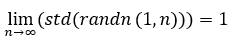and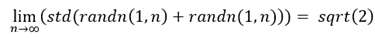The term "signal" actually has two meanings: in the more general sense, it can mean the entire data recording, including the noise and other artifacts, as in the "raw signal" before processing is applied. But it it can also mean only the desirable or important part of the data, the true underlying signal that you seek to measure, as in "signal-to-noise ratio". A fundamental problem in signal measurement is distinguishing the true underlying signal from the noise. You might want to measure the average of the signal over a certain time period or the height of a peak or the area under a peak that occurs in the data. For example, in the absorption spectrum in the right-hand half of Figure 1 in the previous section, the "important" parts of the data are probably the absorption peaks located at 520 and 550 nm. The height or the position of either of those peaks might be considered the signal, depending on the application. In this example, the height of the largest peak is about 0.08 absorbance units. But how to measure the noise?

If you are lucky enough to have a sample and an instrument that are completely stable (except for the random noise), an easy way to isolate and measure the noise is to record two signals m1 and m2 under the same conditions, subtract them point-by-point. Then the
standard deviation of the noise in the original signals is not std(m1-m2) as you might think (that gives too high a value), but rather sqrt((std(m1-m2)2)/2), as shown by a simple derivation based on the rules for mathematical error propagation. The process is demonstrated quantitatively and graphically by the Matlab/Octave script SubtractTwoMeasurements.m (Graphic).

But what if the measurements are not that reproducible or that you had only one recording of that spectrum and no other data?  In that case, you could try to estimate the noise in that single recording, based on the assumption that the visible short-term fluctuations in the signal - the little random wiggles superimposed on the smooth signal - are noise and not part of the true underlying signal. That depends on some knowledge of the origin of the signal and the possible forms it might take. The examples in previous section are absorption spectra of liquid solutions over the wavelength range of 450 nm to 700 nm, which ordinarily exhibit broad smooth peaks with a width of the order of 10 to 100 nm, so the little wiggles must be noise. In this case, those fluctuations amount to a standard deviation of  about 0.001. Often the best way to measure the noise is to locate a section of the signal on the baseline where the signal is flat and to compute the standard deviation in that section. This is easy to do with a computer if the signal is digitized.
The important thing is that you must know enough about the measurement and the data it generates to recognize the kind of signals that is is likely to generate, so you have some hope of knowing what is signal and what is noise.

It's important to appreciate that the standard deviations calculated of a small set of measurements can be much higher or much lower than the actual standard deviation of a larger number of measurements. For example, the Matlab/Octave function randn(1,n), where n is an integer, returns n random numbers that have on average a mean of zero and a standard deviation of 1.00 if n is large. But if n is small, however, standard deviations will be different each time you evaluate that function; for example if n=5, randn(1,5),
the standard deviations might vary randomly from 0.5 to 2 or even more. The is the unavoidable nature of small sets of random numbers: the standard deviation calculated from a small set of numbers is only a very rough approximation to the real underlying standard deviation.

A quick but approximate way to estimate the amplitude of noise visually is the peak-to-peak range, which is the difference between the highest and the lowest values in a region where the signal is flat.
The ratio of peak-to-peak range of n=100 normally-distributed random numbers to its standard deviation is approximately 5, as can be proved by running this line of Matlab/Octave code several times: n=100;rn=randn(1,n);(max(rn)-min(rn))/std(rn). For example, the data on the right half of the figure below, has a peak in the center with a height of about 1.0.  The peak-to-peak noise on the baseline is also about 1.0, so the standard deviation of the noise is about 1/5th of that, or 0.2. However, that ratio varies with the logarithm of n and is closer to 3 when n = 10 and to 9 when n = 100000. In contrast, the standard deviation becomes closer and closer to the true value as n increases. It's better to compute the standard deviation if possible.

In addition to the standard deviation, it's also possible to measure the mean absolute deviation ("mad"). The standard deviation is larger than the mean absolute deviation because the standard deviation weights the large deviation more heavily. For a normally-distributed random variable, the mean absolute deviation is on average 80% of the standard deviation: mad=0.8*std.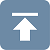The quality of a signal is often expressed quantitatively as the signal-to-noise ratio (S/N ratio), which is the ratio of the true underlying signal amplitude (e.g. the average amplitude or the peak height) to the standard deviation of the noise. Thus the S/N ratio of the spectrum in Figure 1 is about 0.08/0.001 = 80, and the signal in Figure 3 has a  S/N ratio of 1.0/0.2 = 5.  So we would say that the quality of the signal in Figure 1 is better than that in Figure 3 because it has a greater S/N ratio.  Measuring the S/N ratio is much easier if the noise can be measured separately, in the absence of signal. Depending on the type of experiment, it may be possible to acquire readings of the noise alone, for example on a segment of the baseline before or after the occurrence of the signal. However, if the magnitude of the noise depends on the level of the signal, then the experimenter must try to produce a constant signal level to allow measurement of the noise on the signal. In some cases, where you can model the shape of the signal accurately by means of a mathematical function (such as a polynomial or the weighted sum of a number of peak shape functions), the noise may be isolated by subtracting the model from the unsmoothed experimental signal, for example by looking at the residuals in least-squares curve fitting, as in this example. If possible, it's usually better to determine the standard deviation of repeated measurements of the thing that you want to measure (e.g. the peak heights or areas), rather than trying to estimate the noise from a single recording of the data.

Detection limit. The "detection limit" is defined as the smallest signal that you can reliably detect in the presence of noise. In quantitative analysis, it is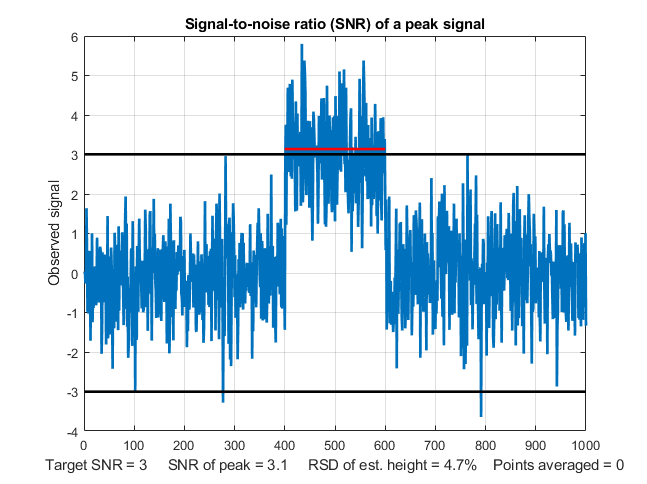usually defined as the concentration that produces the smallest detectable signal (Reference 90). A signal below the detection limit cannot be reliably detected, that is, if the measurement is repeated, the signal will often be "lost in the noise" and reported as zero. A signal above the detection limit will be reliable detected and will seldom or never reported as zero. The most common definition of signal-to-noise ratio at the detection limit is 3. This is illustrated in the figure on the left (created by the Matlab/Octave script SNRdemo.m). This shows a noisy signal in the form of a rectangular pulse. We define the "signal" as the average signal magnitude during the pulse, indicated by the red line, which is 3 ("signal" in line 3 of the script, which you can change). We define the "noise" as the the standard deviation of the random noise on the baseline before and after the pulse, which is about 1.0 (roughly 1/5 of the peak-to-peak baseline noise indicated by the two black horizontal lines). So the signal-to-noise ratio (SNR) in this case is about 3, which is the most common definition of detection limit. This means that this is the lowest signal that can be reliably detected and that signals lower than this should be reported as "undetectable".

But there is a problem. This signal is clearly detectable by eye; in fact, it would be possible to visually detect lower signals than this. How can this be? The answer is "averaging". When you look at this signal, you are unconsciously estimating the average of the data points on the signal pulse and on the base-line, and your visual detection ability in enhanced by this averaging. Without that averaging, looking only at individual data points in the signal, only about half those individual points would meet the SNR=3 criterion. You can see in the graphic above that several points on the signal peak are actually lower that some of the data points on the baseline. But this is not a problem in practice, because any properly written software will include averaging that duplicates the visual averaging that we all do.

In the script SNRdemo.m, the number of points averaged is controlled by the variable "AveragePoints" in line 7. If you set that to 5, the resulting graphic (below on the left)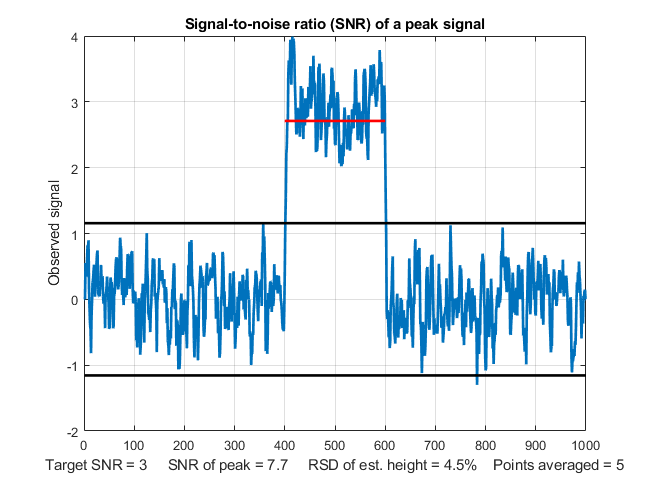shows that all of the signal points are above the highest baseline points. This graphic more accurately represents what we judge when we look at a signal like that in the previous graphic: a clear separation of signal and baseline. The SNR of the peak has improved from 3.1 to 7.7 and the detection limit will be correspondingly reduced. As a rule of thumb, the noise decreases by the roughly the square root of the number of points averaged (sqrt(5)=2.2). Higher values will further improve the SNR and reduce the relative standard deviation of the average signal, but the response time - which is the time it takes for the signal to reach the average value - will become slower and slower as the number of points averaged increases. This is shown by this graphic with 100 points averaged. With a much lower signal, where the SNR is only 1.0, the raw signal is barely detectable visually, but with a 100 point average, the signal precision is good. Digital averaging beats visual averaging in this case.

In SNRdemo.m, the noise is constant and independent of the signal amplitude. In the variant SNRdemoHetero.m, the noise in the signal is directly proportional to the signal level, and as a result the detection limit depends on the constant baseline noise (graphic). In the variant SNRdemoArea.m, it is the peak area that is measured rather than the peak height, and as a result the SNR is improved by the square root of the width of the peak (graphic).

An example of a practical application of a signal like this would be to turn on a warning light or buzzer if the signal ever exceeds a threshold value of 1.5 volts, for the signal illustrated in the figures above. This would not work if you used the raw unaveraged signal in the first figure; there is no threshold value that would never be exceeded by the baseline but always exceeded by the signal.
Only the averaged signal would reliably turn on the alarm above the threshold and never activate it below the threshold.

You will also hear the term "Limit of determination", which is the lowest signal or concentration that achieves a minimum acceptable precision, defined as the relative standard deviation of the signal amplitude. That is defined at much higher signal-to-noise ratio, say 10 or 20, depending on the requirements of your applications.

Averaging such as done here is the simplest form of "smoothing", which is covered in the next chapter.

Ensemble averaging. Onekey thing that really distinguishes signal from noise is that random noise is not the same from one measurement of the signal to the next, whereas the genuine signal is at least partially reproducible. So if the signal can be measured more than once, use can be made of this fact by measuring the signal over and over again, as fast as is practical, and adding up all the measurements point-by-point, then dividing by the number of signals averaged. This is called ensemble averaging, also called "ensemble averaging", and it is one of the most powerful methods for improving signals, when it can be applied. For this to work properly, the noise must be random and the signal must occur at the same time in each repeat.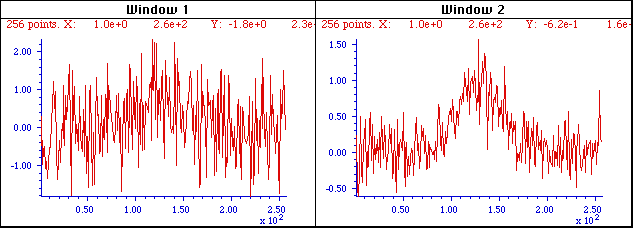Window 1 (left) is a single measurement of a very noisy signal. There is actually a broad peak near the center of this signal, but it is difficult to measure its position, width, and height accurately because the S/N ratio is very poor. Window 2 (right) is the average of 9 repeated measurements of this signal, clearly showing the peak emerging from the noise. The expected improvement in S/N ratio is 3 (the square root of 9). Often it is possible to average hundreds of measurements, resulting in much more substantial improvement. The S/N ratio in the resulting average signal in this example is about 5.

The Matlab/Octave script EnsembleAverageDemo.m demonstrates the technique graphically; click for graphic. Another example is shown in the video animation (EnsembleAverage1.wmv or EnsembleAverageDemo.gif) which shows the ensemble averaging of 1000 repeats of a signal, improving the S/N ratio by about 30 times. (Digitization noise can also be reduced by ensemble averaging, but only if small amounts of random noise are present in, or added to, the signal; see Appendix I).Frequency distribution. Sometimes the signal and the noise can be partly distinguished on the basis of frequency components: for example, the signal may contain mostly low-frequency components and the noise may be located at higher frequencies or spread out over a much wider frequency range. This is the basis of filtering and smoothing.  In the figure above, the peak itself contains mostly low-frequency components, whereas the noise is (apparently) random and is distributed over a much wider frequency range. The frequency of noise is characterized by its frequency spectrum, often described in terms of noise color. White noise is random and has equal power over the range of frequencies. It derives its name from white light, which has equal brightness at all wavelengths in the visible region. The noise in the example signals above and in the upper left quadrant of the figure on the right, is white. In theacoustical domain, white noise sounds like a hiss. In measurement science, white noise is fairly common, For example, quantization noise, Johnson-Nyquist (thermal) noise, photon noise, and the noise made by single-point spikes all have white frequency distributions, and all have in common their origin in discrete quantized instantaneous events, such as the flow of individual electrons or photons.

Noise that has a more low-frequency-weighted character, that is, that has more power at low frequencies than at high frequencies, is often called "pink noise". In the acoustical domain, pink noise sounds more like a roar.  (A commonly-encountered sub-species of pink noise is "1/f noise", where the noise power in inversely proportional to frequency, illustrated in the upper right quadrant of the figure on the right). Pink noise is more troublesome that white noise, because a given standard deviation of pink noise has a greater effect on the accuracy of most measurements than the same standard deviation of white noise (as demonstrated by the Matlab/Octave function noisetest.m which generated the figure on the right). Moreover, the application of smoothing and low-pass filtering to reduce noise is more effective for white noise than for pink noise. When pink noise is present, it is sometimes beneficial to apply modulation techniques, for example optical chopping or wavelength modulation in optical measurements, to convert a direct-current (DC) signal into an alternating current (AC) signal, thereby
increasing the frequency of the signal to a frequency region where the noise is lower. In such cases it is common to use a lock-in amplifier, or the digital equivalent thereof, to measure the amplitude of the signal. Another type of low-frequency weighted noise is Brownian noise, also called "red noise" or "random walk", which has a noise power that is inversely proportional to the square of frequency.  This type of noise is not uncommon in experimental signal and can seriously interfere with accurate signal measurement. See Appendix O: Random walks and baseline correction

Conversely, noise that has more power at high frequencies is called "blue" noise. This type of noise is less commonly encountered in experimental work, but it can occur in processed signals that have been subject to some sort of differentiation process or that have been deconvoluted from some blurring process. Blue noise is easier to reduce by smoothing, and it has less effect on least-squares fits than the equivalent amount of white noise.Dependence on signal amplitude. Noise can also be characterized by the way it varies with the signal amplitude. It may be a constant "background" noise that is independent of the signal amplitude. Or the noise on the background may be very low but may increase with signal amplitude; this is often observed in emission spectroscopy, mass spectroscopy and in the frequency spectra of signals. The fancy names for these two types of behaviors is homoscedastic and heteroscedastic, respectively.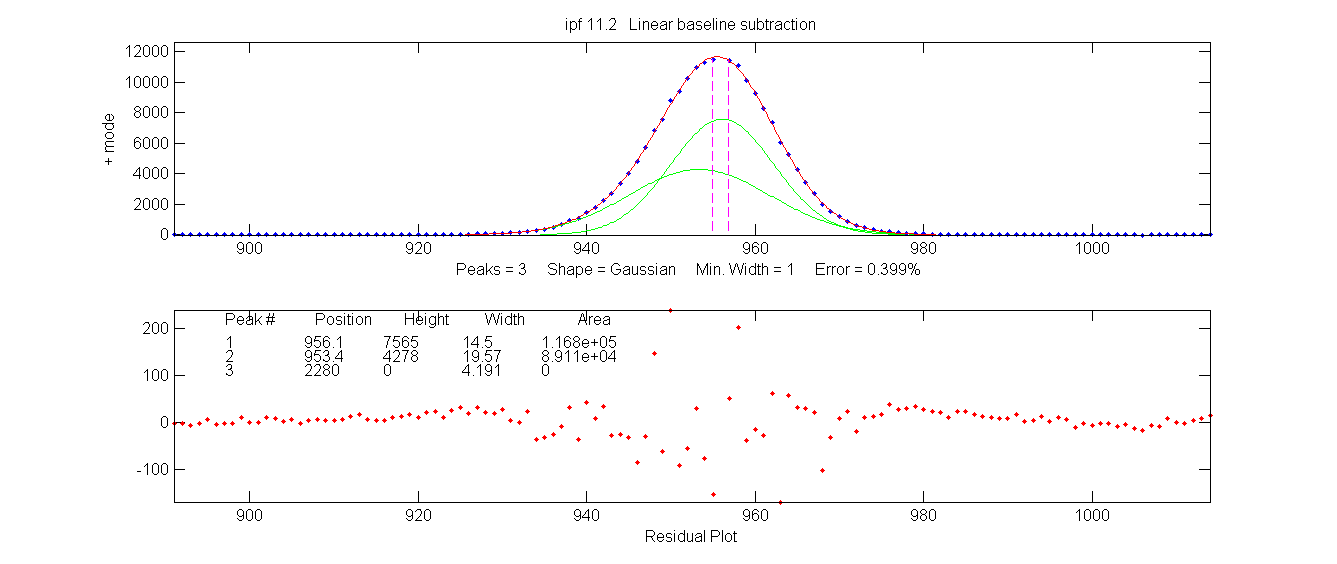One way to observe this is to select a segment of signal over which the signal amplitude varies widely, fit the signal to a polynomial or multiple peak model, and observe how the residuals vary with signal amplitude. The graphic on the left shows a real experimental signal, showing that the residuals from a curve-fitting operation reveals that the noise increases with signal amplitude. Another example shows an example where the noise is almost independent of the signal amplitude.

Often, there is a mix of noises with different behaviors; in optical spectroscopy, three fundamental types of noise are recognized, based on their origin and on how they vary with light intensity: photon noise, detector noise, and flicker (fluctuation) noise. Photon noise (often the limiting noise in instruments that use photo-multiplier detectors) is white and is proportional to the square root of light intensity, and therefore the SNR is proportional to the square root of light intensity and directly proportional to the monochromator slit width. Detector noise (often the limiting noise in instruments that use solid-state photodiode detectors) is independent of the light intensity and therefore the detector SNR is directly proportional to the light intensity and to the square of the monochromator slit width. Flicker noise, caused by light source instability, vibration, sample cell positioning errors, sample turbulence, light scattering by suspended particles, dust, bubbles, etc., is directly proportional to the light intensity (and is usually pink rather than white), so the flicker S/N ratio is not decreased by increasing the slit width. In practice, the total noise observed is likely to be some contribution of all three types of amplitude dependence, as well as a mixture of white and pink noises.

Only in a very few special cases is it possible to eliminate noise completely, so usually you must be satisfied by increasing the S/N ratio as much as possible. The key in any experimental system is to understand the possible sources of noise, break down the system into its parts and measure the noise generated by each part separately, then seek to reduce or compensate for as much of each noise source as possible. For example, in optical spectroscopy, source flicker noise can often be reduced or eliminated by using in feedback stabilization, choosing a better light source, using an internal standard, or specialized instrument designs such as double-beam, dual wavelength, derivative, and wavelength modulation. The effect of photon noise and detector noise can be reduced by increasing the light intensity at the detector or increasing the spectrometer slit width, and electronics noise can sometimes be reduced by cooling or upgrading the detector and/or electronics. Fixed pattern noise in array detectors can be corrected in software.
Only photon noise can be predicted from first principles (e.g. in these spreadsheets that simulate ultraviolet-visible spectrophotometry, fluorescence spectrocopy, and atomic emission spectroscopy.)Probability distribution. Another property that distinguishes random noise is its probability distribution, the function that describes the probability of a random variable falling within a certain range of values.  In physical measurements, the most common distribution is called normal curve (also called as a "bell" or "haystack"curve) and is described by a Gaussian function, y=e^(-(x-mu)^2/(2*sigma^2))/(sqrt(2*mu)*sigma), where mu is the mean (average) value and sigma (
σ ) is the standard deviation. In this distribution, the most common noise errors are small (that is, close to the mean) and the errors become less common the greater their deviation from the mean. So why is this distribution so common? The noise observed in physical measurements is often the balanced sum of many unobserved random events, each of which has some unknown probability distribution related to, for example, the kinetic properties of gases or liquids or to to the quantum mechanical description of fundamental particles such as photons or electrons. But when many such events combine to form the overall variability of an observed quantity, the resulting probability distribution is almost always normal, that is, described by a Gaussian function. This common observation is summed up in the Central Limit Theorem.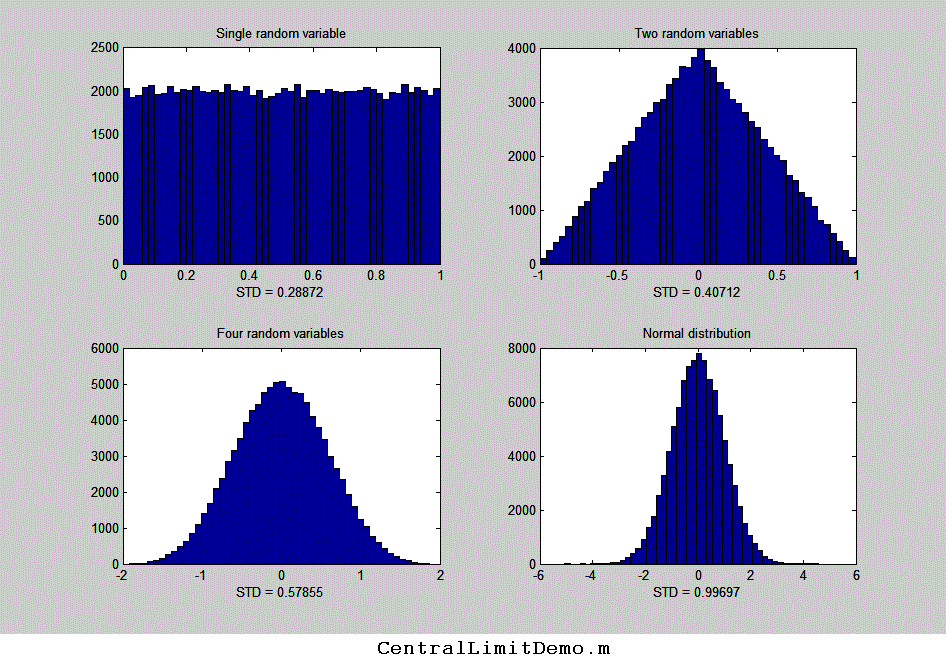This is easily demonstrated by a little simulation. In the example on the left, we start with a set of 100,000 uniformly distributed random numbers that have an equal chance of having any value between certain limits - between 0 and +1 in this case (like the "rand" function in most spreadsheets and Matlab/Octave). The graph in the upper left of the figure shows the probability distribution, called a "histogram", of that random variable. Next, we combine two sets of such independent, uniformly-distributed random variables (changing the signs so that the average remains centered at zero). The result (shown in the graph in the upper right in the figure) has a triangular distribution between -1 and +1, with the highest point at zero, because there are many ways for the difference between two random numbers to be small, but only one way for the difference to be 1 or to -1 (that happens only if one number is exactly zero and the other is exactly 1). Next, we combine four independent random variables (lower left); the resulting distribution has a total range of -2 to +2, but it is even less likely that the result be near 2 or -2 and many more ways for the result to be small, so the distribution is narrower and more rounded, and is already starting to be visually close to a normal Gaussian distribution (shown for reference in the lower right). If we combine more and more independent uniform random variables, the combined probability distribution becomes closer and closer to Gaussian (shown in the bottom right). The Gaussian distribution that we observe here is not forced by prior assumption; rather, it arises naturally. You can download a Matlab script for this simulation from http://terpconnect.umd.edu/~toh/spectrum/CentralLimitDemo.m

Remarkably, the distributions of the individual events hardly matter at all. You could modify the individual distributions in this simulation by including additional functions, such as sqrt(rand), sin(rand), rand^2, log(rand), etc, to obtain other radically non-normal individual distributions. It seems that no matter what the distribution of the single random variable might be, by the time you combine even as few as four of them, the resulting distribution is already visually close to normal. Real world macroscopic observations are often the result of thousands or millions of individual microscopic events, so whatever the probability distributions of the individual events, the combined macroscopic observations approach a normal distribution essentially perfectly. It is on this common adherence to normal distributions that the common statistical procedures are based; the use of the mean, standard deviation
σ , least-squares fits, confidence limits, etc, are all based on the assumption of a normal distribution. Even so, experimental errors and noise are not always normal; sometimes there are very large errors that fall well beyond the "normal" range. They are called "outliers" and they can have a very large effect on the standard deviation σ . In such cases it's common to use the "interquartile range" (IQR), defined as the difference between the upper and lower quartiles, instead of the standard deviation, because the interquartile range is not effected by a few outliers. For a normal distribution, the interquartile range is equal to 1.34896 times the standard deviation. A quick way to check the distribution of a large set of random numbers is to compute both the standard deviation and the interquartile range; if they are roughly equal, the distribution is probably normal; if the standard deviation is much larger, the data set probably contains outliers and the standard deviation without the outliers can be better estimated by dividing the interquartile range by 1.34896.

The importance of the normal distribution is that if you know the standard deviation σ of some measured value, then you can predict the likelihood that your result might be in error by a certain amount.  About 68% of values drawn from a normal distribution are within one σ away from the mean; 95% of the values lie within 2σ; and 99.7% are within 3σ. This is known as the "68-95-99.7" or the 3-sigma rule. But the real practical problem is that standard deviations are hard to measure accurately unless you have large numbers of samples. See The Law of Large Numbers.

It important to understand that the three characteristics of noise just discussed in the paragraphs above - the frequency distribution, the amplitude distribution, and the signal dependence - are mutually independent; a noise may in principle have any combination of those properties.Visual animation of ensemble averaging. This 17-second video (EnsembleAverage1.wmv) demonstrates the ensemble averaging of 1000 repeats of a signal with a very poor S/N ratio. The signal itself consists of three peaks located at x = 50, 100, and 150, with peak heights 1, 2, and 3 units. These signal peaks are buried in random noise whose standard deviation is 10. Thus the S/N ratio of the smallest peaks is 0.1, which is far too low to even see a signal, much less measure it. The video shows the accumulating average signal as 1000 measurements of the signal are performed. At the end, the noise is reduced (on average) by the square root of 1000 (about 32), so that the S/N ratio of the smallest peaks ends up being about 3, just enough to detect the presence of a peak reliably. Click here to download the video (2 MBytes) in WMV format. (This demonstration was created in Matlab 6.5. If you have access to that software, you may download the original m-file, EnsembleAverage.zip).Popular spreadsheets, such as Excel or Open Office Calc, have built-in functions that can be used for calculating, measuring and plotting signals and noise. For example, the cell formula for one point on a Gaussian peak is amplitude*EXP(-1*((x-position)/(0.6005615*width))^2), where 'amplitude' is the maximum peak height, 'position' is the location of the maximum on the x-axis, 'width' is the full width at half-maximum (FWHM) of the peak (which is equal to sigma times 2.355), and 'x' is the value of the independent variable at that point. The cell formula for a Lorentzian peak is amplitude/(1+((x-position)/(0.5*width))^2). Other useful functions include AVERAGE, MAX, MIN, STDEV,  RAND, and QUARTILE.

Most spreadsheets have only a uniformly-distributed random number function (RAND) and not a normally-distributed random number function, but it's much more realistic to simulate errors that are normally distributed. In that case it's convenient to make use of the Central Limit Theorem to create approximately normally distributed random numbers by combining several RAND functions, for example, the expression
sqrt(3)*(RAND()-RAND()+RAND()-RAND()) creates nearly normal random numbers with a mean of zero, a standard deviation very close to 1, and a maximum range of plus or minus 4. I use this trick in spreadsheet models that simulate the operation of analytical instruments.  (The expression sqrt(2)*(rand()-rand()+rand()-rand()+rand()-rand()) works similarly, but has a larger maximum range of plus or minus 5). To create random numbers with a standard deviation other that 1, simply multiply by that number; to create random numbers with an average other that zero, simply add that number.

The interquartile range (IQR) can be calculated in a spreadsheet by subtracting the third quartile from the first (e.g.
QUARTILE(B7:B504,3)-QUARTILE(B7:B504,1)). The spreadsheets RandomNumbers.xls, for Excel, and RandomNumbers.ods, for OpenOffice, (screen image), and the Matlab/Octave script RANDtoRANDN.m, demonstrate these facts. The same technique is used in the spreadsheet SimulatedSignal6Gaussian.xlsx , which computes and plots a simulated signal consisting of up to 6 overlapping Gaussian bands plus random white noise.

Matlab and Octavehave built-in functions that can be used for for calculating, measuring and plotting signals and noise, including mean, max, min, std, kurtosis, skewness, plot, hist, histfit, rand, and randn. Just type "help" and the function name at the command >> prompt, e.g. "help mean".  Most of these  functions apply to vectors and matrices as well as scalar variables. For example, if you have a series of results in a vector variable 'y', mean(y) returns the average and std(y) returns the standard deviation of all the values in y. For vectors, std computes sqrt(mean(y.^2)). You can subtract a scalar number from a vector (for example, v = v-min(v) sets the lowest value of vector v to zero).  If you have a set of signals in the rows of a matrix S, where each column represents the value of each signal at the same value of the independent variable (e.g. time), you can compute the ensemble average of those signals just by typing "mean(S)", which computes the mean of each column of S. Note that function and variable names are case sensitive.

As an example of the "randn" function in Matlab/Octave, it is used here to generate 100 normally-distributed random numbers, then the "hist" function computes the "histogram" (probability distribution) of those random numbers, then the downloadable function peakfit.m fits a Gaussian function (plotted with a red line) to that distribution:

>>[N,X]=hist(randn(size(1:100)));
>> peakfit([X;N])
;

If you change the 100 to 1000 or a higher number, the distribution becomes
closer and closer to a perfect Gaussian and its peak falls closer to 0.00. The "randn" function is useful in signal processing for predicting the uncertainty of measurements in the presence of random noise, for example by using the Monte Carlo or the bootstrap methods that will be described in a later section.
(You can copy and paste, or drag and drop, these two lines of code into the Matlab or Octave editor or into the command line and press Enter to execute it).

Here is an MP4 animation that demonstrates the gradual emergence of a Gaussian normal distribution and the number of samples increase from 2 to 1000. Note how many samples it takes before the normal distribution is well-formed.In Python, these basic math functions are similar: len(d), max(d), min(d), abs(d), sum(d), and, after importing numpy as np, np.sum(d), np.mean(d), np.std(d), np.sqrt(d). The random function is np.random.rand(d). The variable d can be a scalar, vector, or matrix.

The difference between scripts and functions.

If you find that you are writing or copying and pasting the same series of Matlab commands repeatedly, consider writing a script or a function that will save your code to the computer so you can use it again more easily. It is extremely handy to create your own user-defined scripts and functions in Matlab or Octave to automate commonly used algorithms.

Scripts and functions are just simple text files saved with the ".m" file extension to the file name. The difference between a script and a function is that a function definition begins with the word 'function'; a script is just any list of Matlab commands and statements. For a script, all the variables defined and used are listed in Matlab's Workspace window and shared with other scripts. For a function, on the other hand, the variables are internal and private to that function; values can be passed to the function through the input variables (also called arguments), and values can be passed from the function through the output variables, which are both defined in the first line of the function definition.

[output variables] = FunctionName(input variables)

That means that functions are a great way to package chunks of code that perform useful operations in a form that can be used as components in other scripts and functions without worrying that the internal variable names within the function will conflict and cause errors. When you write a function, it is saved to the computer and can be called again on that computer, just like the built-in functions that came with Matlab. For an example of a very simple function, look at the code for rsd.m.

function relstddev=rsd(x)
% Relative standard deviation of vector x
relstddev=std(x)./mean(x);

Scripts and functions can call other functions; scripts must have those functions in the Matlab path; functions, on the other hand, can have all their required sub-functions defined within the main function itself and thus can be self-contained. If you write a script or function that calls one or more of your custom functions, and you send it to someone else, be sure to include all the custom functions that it calls. (It is best to make all your functions self-contained with all required sub-functions included).

If you run one of my scripts and get an error message that says, "Undefined function...", you need to download the specified function from http://tinyurl.com/cey8rwh and place it in the Matlab/Octave path. Note: in Matlab R2016b or later, you CAN include functions within scripts; just place them at the end of the script and add an additional "end" statement to each. (see https://www.mathworks.com/help/matlab/matlab_prog/local-functions-in-scripts.html.

For writing or editing scripts and functions, Matlab and the latest version of Octave have an internal editor. For an explanation of a function and a simple worked example, type "help function" at the command prompt. When you are writing your own functions or scripts, you should always add lots of "comment lines" (beginning with the character %) that explain what is going on. You will be glad you did later. The first group of comment lines, up to the first blank line that does not begin with a %, are used as the "help file" for that script or function. Typing "help ___", where ___ is the name of the function, displays the comment lines for that function or script in the command window, just as it does for the built-in functions and scripts. This will make your scripts and functions much easier to understand and use, both by other people and by yourself in the future. Resist the temptation to skip this. As you develop custom functions for your own work, you will be developing a "toolkit" that will become very useful to your students or co-workers, or even to yourself in the future, if you use comments liberally.

Here's a very handy helper: when you type a function name into the Matlab editor, if you pause for a moment after typing the open parenthesis immediately after the function name, Matlab will display a pop-up listing all the possible input arguments as a reminder. This works even for downloaded functions and for any new functions that you yourself create. It's especially handy when there are so many possible input arguments that it's hard to remember all of them. The popup stays on the screen as you type, highlighting each argument in turn:This feature is easily overlooked, but it's very handy. Clicking on
More Help... on the right displays the help for that function in a separate window.Some examples of my Matlab/Octave user-defined functions related to signals and noise that you can download and use are: stdev.m, a standard deviation function that works in both Matlab and in Octave; rsd.m, the relative standard deviation; halfwidth.m for measuring the full width at half maximum of smooth peaks; plotit.m, an easy-to-use function for plotting and fitting x,y data in matrices or in separate vectors; functions for peak shapes commonly encountered in analytical chemistry such as GaussianLorentzian, lognormal, Pearson 5, exponentially-broadened Gaussian, exponentially-broadened Lorentzian, exponential pulse, sigmoidbifurcated Lorentzianpeakfunction.m, a function that generates any of those peak types specified by number. ShapeDemo demonstrates the 12 basic peak shapes graphically, showing the variable-shape peaks as multiple lines. There are functions for different types of random noise (white noise, pink noise, blue noise, proportional noise, and square root noise), a function that applies exponential broadening (ExpBroaden.m), a function that computes the interquartile range (IQrange.m), a function that estimates the standard deviation of a distribution with outliers by computing the interquartile range and dividing it by 1.34896 (stdiqr.m); a function that removes "not-a-number" entries from vectors (rmnan.m), and a function that returns the index and the value of the element of vector x that is closest to a particular value (val2ind.m). These functions can be useful in modeling and simulating analytical signals and testing measurement techniques. You can click or ctrl-click on these links to inspect the code or you can right-click and select "Save link as..." to download them to your computer. Once you have downloaded those functions and placed them in the "path", you can use them just like any other built-in function. For example, you can plot a simulated Gaussian peak with white noise by typing: x=[1:256]; y=gaussian(x,128,64) + whitenoise(x); plot(x,y). The script plotting.m uses the gaussian.m function to demonstrate the distinction between the height, position, and width of a Gaussian curve. The script SignalGenerator.m calls several of these downloadable functions to create and plot a realistic computer-generated signal with multiple peaks on a variable baseline plus variable random noise; you might try to modify the variables in the indicated places to make it look like your type of data. These functions have been developed and tested in Matlab 7.8 (R2009a), 8.1 (R2013a), 9.3 (R2017b home version), R2018b Student version, and in R2020b update 3. Almost all of these functions will work in the latest version of Octave without change.  For a complete list of  downloadable functions and scripts developed for this project, see functions.html.

The Matlab/Octave script EnsembleAverageDemo.m demonstrates ensemble averaging to improved the S/N ratio of a very noisy signal. Click for graphic. The script requires the "gaussian.m" function to be downloaded and placed in the Matlab/Octave path, or you can use another peak shape function, such as lorentzian.m or rectanglepulse.m.

The Matlab/Octave function noisetest.m demonstrates the appearance and effect of different noise types. It plots Gaussian peaks with four different types of added noise: constant white noise, constant pink (1/f) noise, proportional white noise, and square-root white noise, then fits a Gaussian to each noisy data set and computes the average and the standard deviation of the peak height, position, width and area for each noise type. Type "help noisetest" at the command prompt. The  Matlab/Octave script SubtractTwoMeasurements.m demonstrates the technique of subtracting two separate measurements of a waveform to extract the random noise (but it works only if the signal is stable, except for the noise). Graphic.

iSignal isa downloadable Matlab function that can plot signals with pan and zoom controls, measure signal and noise amplitudes in selected regions of the signal, and compute the S/N ratio of peaks. It's operated by simple key presses. Other capabilities of iSignal include smoothing, differentiation, peak sharpening, and least-squares peak measurement. View the code here or download the ZIP file with sample data for testing. (Octave users require a separate version: isignaloctave.m).

For signals that contain repetitive waveform patterns occurring in one continuous signal, with nominally the same shape except for noise, the interactive peak detector function iPeak has an ensemble averaging function (Shift-E) can compute the average of all the repeating waveforms. It works by detecting a single reference peak in each repeat waveform in order to synchronize the repeats (and therefore does not require that the repeats be equally spaced or synchronized to an external reference signal). To use this function, first adjust the peak detection controls to detect only one peak in each repeat pattern, then zoom in to isolate any one of those repeat patterns, and then press Shift-E. The average waveform is displayed in Figure 2 and saved as EnsembleAverage.mat" in the current directory. See iPeakEnsembleAverageDemo.m for a demonstration.

See
Appendix S: Measuring the Signal-to-Noise Ratio of Complex Signals for more examples of S/N ratio in Matlab/Octave.This page is part of "A Pragmatic Introduction to Signal Processing", created and maintained by Prof. Tom O'Haver , Department of Chemistry and Biochemistry, The University of Maryland at College Park. Comments, suggestions and questions should be directed to Prof. O'Haver at toh@umd.edu. Updated December, 2021.
Unique visits since June 17, 2009: